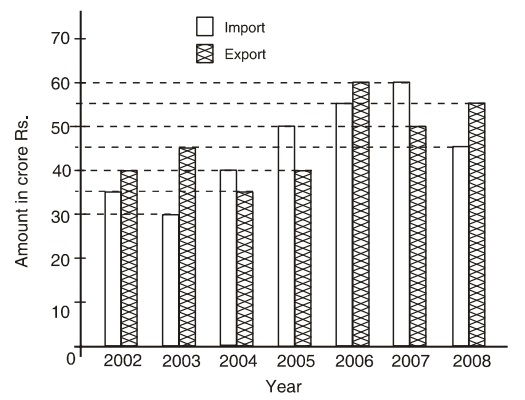Home » Data Interpretation » Bar graph » Question

#### Data Interpretation

Direction: Study the following graph carefully to answer the questions given below it.
Imports and Exports of spare parts by an automobile company over the given years.1. What is the ratio of total imports to the total exports for all the given years together ?
1. 31 : 25
2. 35 : 31
3. 63 : 65
4. 65 : 63
##### Correct Option: C

Total import = 35 + 30 + 40 + 50 + 55 + 60 + 45 = Rs. 315 crores
Total export = 40 + 55 + 35 + 40 + 60 + 50 + 55 = Rs. 335 crores
Since, their ratio = 315 : 335 = 63 : 65